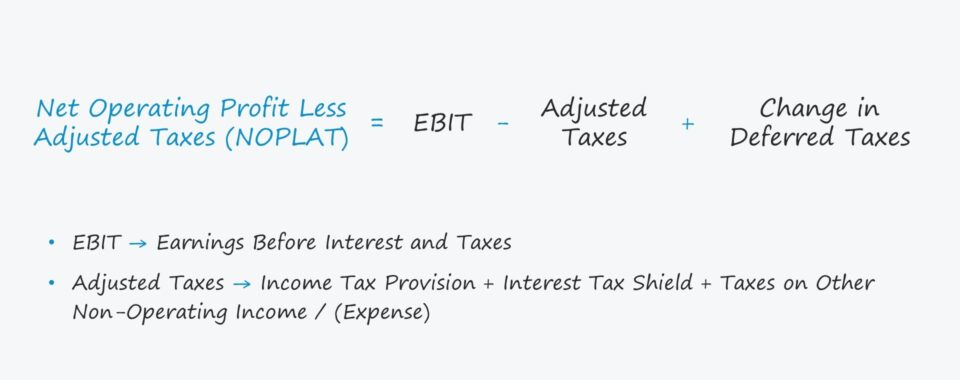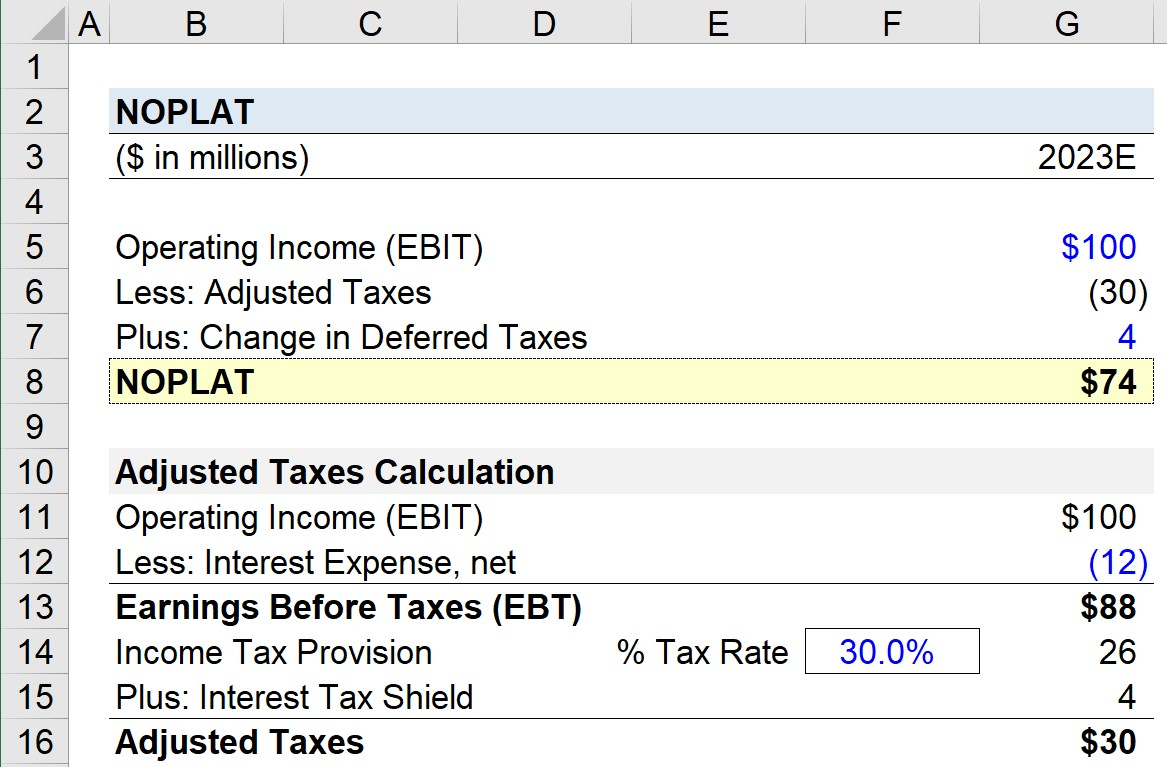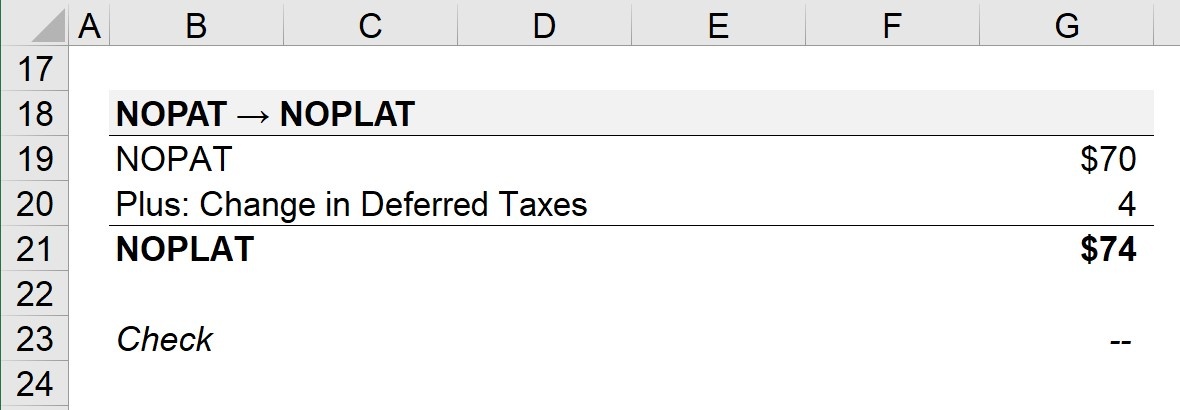Welcome to Wall Street Prep! Use code at checkout for 15% off.# NOPLAT

Guide to Understanding NOPLAT## How to Calculate NOPLAT?

A company’s net operating profit less adjusted taxes (NOPLAT) calculates a company’s operating income (i.e. EBIT) after adjusting for taxes.

By starting with EBIT – a capital structure-neutral financial metric – NOPLAT is not affected by the net interest expense of a company.

Interest is a non-core part of a company’s operations and is impacted by discretionary decisions surrounding debt and equity financing, i.e. the proportion of debt within the company’s total capitalization.

When the capital structure decisions unique to the specific company are removed, the metric becomes better suited for the following:

Once the operating income is calculated, the next step is to tax-affect it using the company’s tax rate.

The rationale for not using the actual tax expense value is because interest – or more specifically, the interest tax shield – impacts the taxes owed.

Since NOPLAT is attempting to reflect the taxes owed on core operations, as opposed to non-core operations, we multiply EBIT by one minus the tax rate.

In the final step, adjustments are made to NOPLAT to factor in any existing deferred taxes, i.e. to add back the taxes overpaid (or underpaid). Deferred taxes are NOT actually paid in cash, so these non-cash charges are treated as an add-back.

## NOPLAT Formula

The formula for calculating NOPLAT equals operating income (EBIT) subtracted by adjusted taxes, with a positive adjustment for any change in deferred taxes.

NOPLAT = EBITAdjusted Taxes + Change in Deferred Taxes

Where:

• Adjusted Taxes = Income Tax Provision + Interest Tax Shield + Taxes on Other Non-Operating Income / (Expense)

## NOPLAT vs. NOPAT: What is the Difference?

NOPLAT and NOPAT are often used interchangeably, although the NOPAT metric is far more prevalent in practice.

NOPLAT is taught in the Chartered Financial Analyst (CFA) exam curriculum and also appears in the book “Valuation: Measuring and Managing the Value of Companies” published by McKinsey.

For the most part, NOPAT and NOPLAT are conceptually very similar, except the latter takes into account deferred tax liabilities (DTLs) or deferred tax assets (DTAs) directly.

But do note that NOPAT does not necessarily neglect those DTLs / DTAs entirely, i.e. the projected tax rate assumption could be indirectly normalized with consideration for the company’s deferred taxes.

In short, if a company carries no deferred taxes, then NOPAT will be equal to NOPLAT.

## NOPLAT Calculator

We’ll now move to a modeling exercise, which you can access by filling out the form below.Submitting...

## Step 1. Pre-Tax Income Calculation (EBT)

Suppose you’re tasked with forecasting a company’s future cash flows to create an unlevered discounted cash flow (DCF) model.

For our hypothetical scenario, we’ll assume that the company is projected to generate \$100 million in operating income (EBIT) for the next fiscal year, 2023.

• Operating Income (EBIT) = \$100 million

We need to deduct the adjusted taxes from EBIT, which we’ll calculate separately below.

To start, we’ll bring down our EBIT value by linking to it and then assuming interest expense of \$12 million.

• Interest Expense, net = \$12 million

If we subtract the interest from EBIT, we’re left with \$88 million of earnings before taxes (EBT), i.e. the pre-tax income.

• EBT = \$100 million – \$12 million = \$88 million

## Step 2. Adjusted Taxes and NOPLAT Calculation

Upon multiplying our company’s EBT by the tax rate assumption of 30% – which is above the normalized forward-looking tax rate of the company since more taxes were recorded than actually paid – the income tax provision amounts to \$26 million.

The \$26 million is the tax expense amount that would appear on the income statement, but we must adjust for the interest tax shield, which we’ll calculate by tax-affecting the interest expense.

• Tax Rate = 30%
• Income Tax Provision = \$88 million × 30% = \$26 million
• Interest Tax Shield = \$12 million × 30% = \$4 million

The calculation of adjusted taxes is now complete and will be subsequently linked to the earlier section.

• Adjusted Taxes = \$26 million + \$4 million = \$30 million

Thus far, we have determined the values for EBIT and adjusted taxes, so the only remaining input is the change in deferred taxes, which we’ll assume to be \$4 million.

If we subtract the adjusted taxes from EBIT and add back the change in deferred taxes, we arrive at a NOPLAT of \$74 million.

• NOPLAT = \$100 million – \$30 million + \$4 million = \$74 million## Step 3. NOPAT to NOPLAT Calculation Analysis Example

In the final part of our modeling exercise, we’ll calculate NOPLAT from NOPAT.

The approach we’ll use here is simpler and results in the same value, but is less intuitive for understanding NOPLAT the first time.

In order to calculate NOPAT, we’ll multiply EBIT by one less our tax rate assumption.

• NOPAT = \$100 million × (1 – 30.0%) = \$70 million

The only difference between NOPAT vs. NOPLAT is the adjustment for deferred taxes, so our last step is to add back the change in deferred taxes.

• NOPLAT = \$70 million + \$4 million = \$74 million

Therefore, in either approach, the NOPLAT for our company in 2023 is confirmed to be \$74 million.Step-by-Step Online Course

### Everything You Need To Master Financial Modeling

Enroll in The Premium Package: Learn Financial Statement Modeling, DCF, M&A, LBO and Comps. The same training program used at top investment banks.